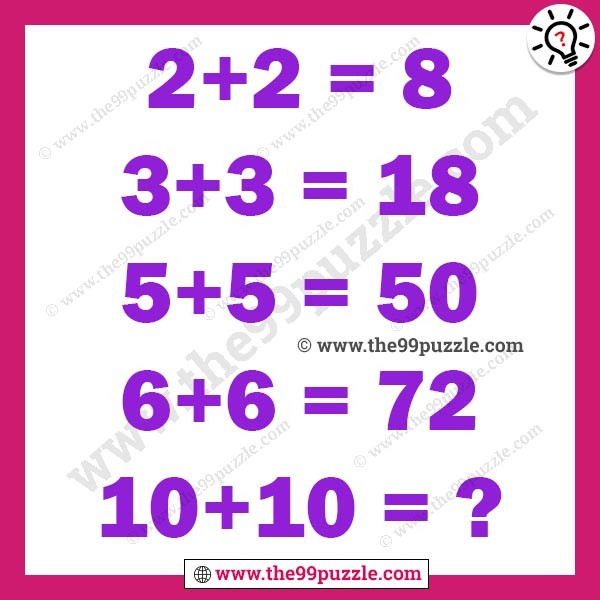# Mind challenging maths logical questions and answers – Puzz161

Mind challenging maths logical can solve both kids and adults all grade students. 99% of people fail to answer this simple math logic equation. If you are intelligent people you can easily solve these puzzles. Challenge your logical mind to solve this puzzle. Most intelligent people fail to answer it. If you are a pro in mathematics you can solve the last equation of this puzzle quickly.

2+2=8

3+3=18

5+5=50

6+6=72

10+10=?###### Explanation:

2+2=(2+2)×2=8

3+3=(3+3)×3=18

5+5=(5+5)×5=50

6+6=(6+6)×6=72

10+10=(10+10)×10=200